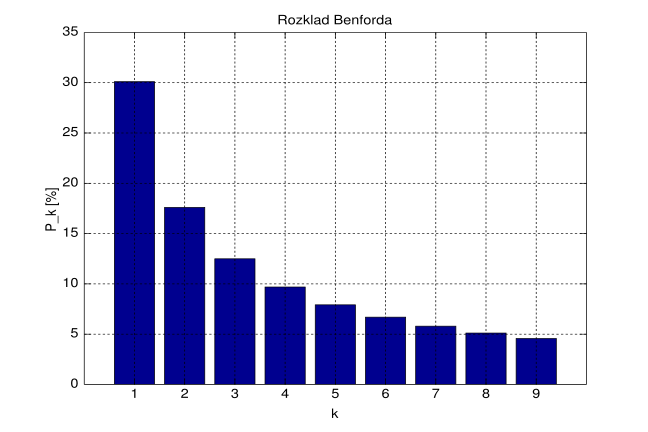## Want to keep learning?

This content is taken from the UNSW Sydney's online course, Maths for Humans: Inverse Relations and Power Laws. Join the course to learn more.
2.5

## UNSW SydneyWhat is the first digit?

# Benford's law

It turns out that in many different contexts, when we look at the first significant digit of numbers appearing in various kinds of data, we find that the digits $$\normalsize{1,2,3,4,5,6,7,8}$$ and $$\normalsize{9}$$ do not all occur with equal frequency. The smaller digits are much more frequent than the larger ones, and there appears to be a systematic relationship between the size of the digit and its likelihood of occurring as a first digit.

In this step we will

• see how this law, while not an inverse relationship, is closely connected to $$\normalsize{y=1/x}$$

• get an idea how this law can be applied in criminal and economic investigations.

## Simon Newcomb’s curious observation, and Frank Benford’s investigations

When we look at numbers that appear in real-life data, we find that the digit $$\normalsize{1}$$ occurs much more often than the digit $$\normalsize{9}$$ as the first digit: in fact it occurs more than $$\normalsize{6}$$ times as often. This was first observed by Simon Newcomb, an American astronomer in 1881.

It was put on the map in 1938 by the American physicist Frank Benford, who investigated thousands of occurrences of the law in a wide range of seemingly unrelated examples, from surface areas of rivers, population tables, physical constants, molecular weights, mathematical handbook entries, and even numbers contained in an issue of Reader’s Digest.

## Relative frequencies of first digits

Here are the relative frequencies of the familiar nine non-zero digits as the first-most digit of numbers

$$\normalsize{d}$$ $$\normalsize{P(d)}$$
1 30.1%
2 17.6%
3 12.5%
4 9.7%
5 7.9%
6 6.7%
7 5.8%
8 5.1%
9 4.6%Q1 (M): Verify that $$\normalsize{P(1)=P(2)+P(3)}$$, that $$\normalsize{P(2)=P(4)+P(5)}$$, and that $$\normalsize{P(3)=P(6)+P(7)}$$. Can you find another such relation? Does this suggest to you anything that we have already looked at in this course?

Q2 (M): Can you find any other expression that allows one to write $$\normalsize{P(1)}$$ as a sum of higher $$\normalsize{P(d)}$$’s?

## Relation with the $$\normalsize{y=1/x}$$ function

While the graph of $$\normalsize{P(d)}$$ as a function of $$\normalsize{d}$$ might look like an inverse proportionality, it is not an inverse proportionality. But remarkably it is not very far from it!

In the following figure we see the approximate areas of equally spaced regions under $$\normalsize{y=1/x}$$ from $$\normalsize{x=1}$$ to $$\normalsize{x=10}$$. The total area is approximately $$\normalsize{2.303}$$.Now in the following diagram, we see the relative sizes of these areas, where we have normalized by dividing by the total area from $$\normalsize{x=1}$$ to $$\normalsize{x=10}$$, namely $$\normalsize{2.303}$$. Remarkably, we see exactly the numbers appearing in Benford’s law!Another way of saying this, using the $$\normalsize{\ln\;x}$$ function representing the area under $$\normalsize{y=1/x}$$ from $$\normalsize{x=1}$$ to $$\normalsize{x}$$, is that the probability of the digit $$\normalsize{d=1,2,3,...9}$$ is just

$\Large{P(d)=\frac{\ln (d+1)-\ln\, d}{\ln 10}}.$

## Applications to detective work

Investigators can use Benford’s law to suggest when data has been manipulated or made up. If you cook up the figures in your tax return, chances are your distribution of digits will be more towards uniform than Benford’s law would suggest. So a computer can sniff out suspect-looking data just by counting digits!

## Applications to modern geopolitics

Here is the abstract from the paper Fact and Fiction in EU-Government Data from the German Economic Review, published in 2011.

To detect manipulations or fraud in accounting data, auditors have successfully used Benford’s law as part of their fraud detection processes. Benford’s law proposes a distribution for first digits of numbers in naturally occurring data. Government accounting and statistics are similar in nature to financial accounting. In the European Union (EU), there is pressure to comply with the Stability and Growth Pact criteria. Therefore, like firms, governments might try to make their economic situation seem better. In this paper, we use a Benford test to investigate the quality of macroeconomic data relevant to the deficit criteria reported to Eurostat by the EU member states. We find that the data reported by Greece shows the greatest deviation from Benford’s law among all euro states.

Fact and Fiction in EU-Governmental Economic Data(German Economic Review 12(3): 243–255)

## Discussion

What do you think about Benford’s law? You might like to have a look at some data from some books, or the internet, and make a little tally, and tell us if Benford’s law seems to hold.

A1. It is also true that $$\normalsize{P(4)=P(8)+P(9)}$$. This reminds us of the dilation property of areas under the graph of $$\normalsize{y=1/x}$$.
A2. (M): How about $$\normalsize{P(1)=P(4)+P(5)+P(6)+P(7)}$$.